The OpenFOAM Foundation
reducedUnits Class Reference

## Public Member Functions

reducedUnits ()
Construct with no argument, uses default values: More...

reducedUnits (scalar refLength, scalar refTime, scalar refMass)
Construct from components. More...

reducedUnits (const IOdictionary &reducedUnitsDict)
Construct from dictionary. More...

~reducedUnits ()
Destructor. More...

void setRefValues (scalar refLength, scalar refTime, scalar refMass)

void setRefValues (const IOdictionary &reducedUnitsDict)

scalar refLength () const

scalar refTime () const

scalar refMass () const

scalar refTemp () const

scalar refEnergy () const

scalar refForce () const

scalar refVelocity () const

scalar refVolume () const

scalar refPressure () const

scalar refMassDensity () const

scalar refNumberDensity () const

## Static Public Attributes

static const scalar kb = 1.3806504e-23
Static data someStaticData. More...

## Friends

Ostreamoperator<< (Ostream &, const reducedUnits &)

## Detailed Description

Source files

Definition at line 57 of file reducedUnits.H.

## Constructor & Destructor Documentation

 reducedUnits ( )

Construct with no argument, uses default values:

length = 1nm mass = 1.660538782e-27kg (unified atomic mass unit) temperature = 1K (therefore, energy = 1*kb)

Definition at line 73 of file reducedUnits.C.

 reducedUnits ( scalar refLength, scalar refTime, scalar refMass )

Construct from components.

Definition at line 84 of file reducedUnits.C.

 reducedUnits ( const IOdictionary & reducedUnitsDict )

Construct from dictionary.

Definition at line 98 of file reducedUnits.C.

References reducedUnits::setRefValues().

Here is the call graph for this function: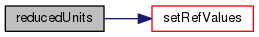~reducedUnits ( )

Destructor.

Definition at line 110 of file reducedUnits.C.

References reducedUnits::setRefValues().

Here is the call graph for this function: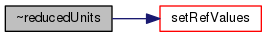## Member Function Documentation

 void setRefValues ( scalar refLength, scalar refTime, scalar refMass )

Definition at line 117 of file reducedUnits.C.

Referenced by reducedUnits::reducedUnits(), and reducedUnits::~reducedUnits().

Here is the call graph for this function: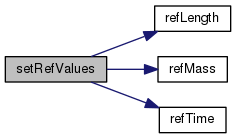Here is the caller graph for this function:void setRefValues ( const IOdictionary & reducedUnitsDict )

Definition at line 134 of file reducedUnits.C.

Here is the call graph for this function: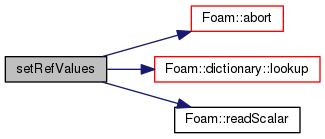Foam::scalar refLength ( ) const
inline

Definition at line 28 of file reducedUnitsI.H.

Referenced by Foam::operator<<(), and reducedUnits::setRefValues().

Here is the caller graph for this function: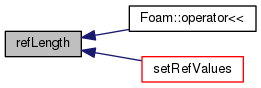Foam::scalar refTime ( ) const
inline

Definition at line 34 of file reducedUnitsI.H.

Referenced by Foam::operator<<(), and reducedUnits::setRefValues().

Here is the caller graph for this function: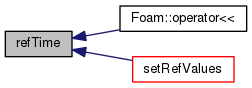Foam::scalar refMass ( ) const
inline

Definition at line 40 of file reducedUnitsI.H.

Referenced by Foam::operator<<(), and reducedUnits::setRefValues().

Here is the caller graph for this function: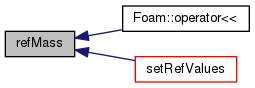Foam::scalar refTemp ( ) const
inline

Definition at line 46 of file reducedUnitsI.H.

Referenced by Foam::operator<<().

Here is the caller graph for this function: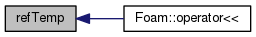Foam::scalar refEnergy ( ) const
inline

Definition at line 52 of file reducedUnitsI.H.

Referenced by Foam::operator<<().

Here is the caller graph for this function: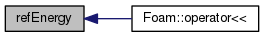Foam::scalar refForce ( ) const
inline

Definition at line 58 of file reducedUnitsI.H.

Referenced by Foam::operator<<().

Here is the caller graph for this function: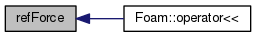Foam::scalar refVelocity ( ) const
inline

Definition at line 64 of file reducedUnitsI.H.

Referenced by Foam::operator<<().

Here is the caller graph for this function: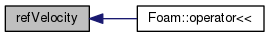Foam::scalar refVolume ( ) const
inline

Definition at line 70 of file reducedUnitsI.H.

Referenced by Foam::operator<<().

Here is the caller graph for this function: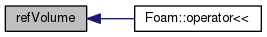Foam::scalar refPressure ( ) const
inline

Definition at line 76 of file reducedUnitsI.H.

Referenced by Foam::operator<<().

Here is the caller graph for this function: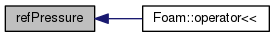Foam::scalar refMassDensity ( ) const
inline

Definition at line 82 of file reducedUnitsI.H.

Referenced by Foam::operator<<().

Here is the caller graph for this function: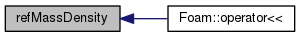Foam::scalar refNumberDensity ( ) const
inline

Definition at line 88 of file reducedUnitsI.H.

Referenced by Foam::operator<<().

Here is the caller graph for this function: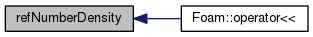## Friends And Related Function Documentation

 Ostream& operator<< ( Ostream & , const reducedUnits & )
friend

## Member Data Documentation

 const Foam::scalar kb = 1.3806504e-23
static

Static data someStaticData.

Definition at line 106 of file reducedUnits.H.

The documentation for this class was generated from the following files: